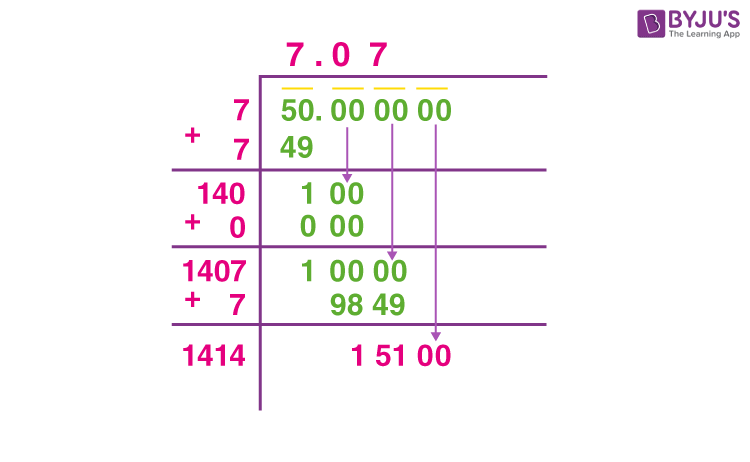# How to find square root of 50 by long division method?

Follow the below steps to find the square root using long division method.

1. Write number 50 as 50.000000
2. Take the number whose square is less than or equal to 50. Hence, 72= 49 and 49<50
3. Divide 50 by 7, such that we get quotient equal to 7 and remainder is 1.
4. Add 7 to the quotient,i.e. 7+7 = 14 and write it down.
5. Drag and drop two zeros from the dividend next to the remainder 1 and add 7 to the quotient
6. Again take such a number that can be combined with 14 and multiplied with itself to get a value less than equal to 100.
7. So, 140 multiplied by 0 will give 0 and remainder equal to 100
8. Now add 0 to the previous quotient and write it down, as shown.
9. Again drag and drop the next two zeros from the top, to make the next dividend equal to 10000.
10. Now, take a value  to combine with 140 and multiplied with itself to get a number less than 10000. 1407 x 7 = 9849 and remainder = 151
11. We have got the square root of 50 up to two places of decimal.
12. Repeat the method to find the square root with more accuracy.(3)(0)